cancel
Showing results for
Did you mean:

## A/B Testing Analysis with Incorta PySparkEmployee Alumni

## Overview

This article helps you begin with A/B testing analysis with Incorta.  The goal is to show how to implement the A/B Test in PySpark codes using Incorta Materialized View.

The code included can also be used to show how to use PySpark UDF (User Defined Function)

The Spark UDF and the approach developed can be used in your A/B Test projects.

## What is A/B testing?

A/B testing is a randomized experimental process in which two or more versions of a variable are presented to users, and the experiment result will determine whether the new version has an significant impact and drives business metrics.

## Case Study

This article will go through a case that a change to a website's product page that aims to increase customer purchases and drive business metrics.

### Data Set

The dataset comes from Kaggle:

Here is a preview of the data after you upload the dataset to Incorta.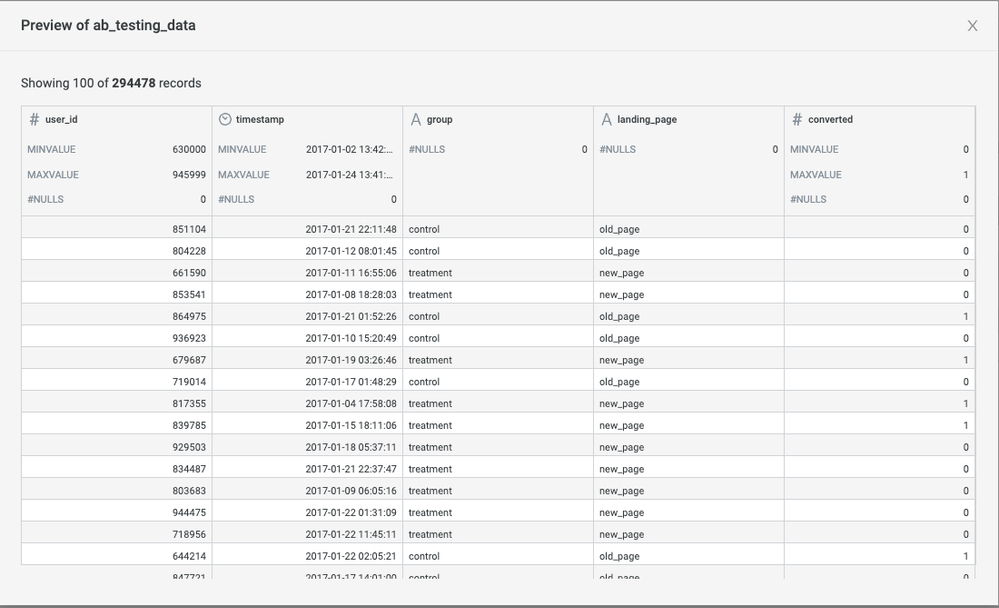The dataset has five columns:

• user_id: The user id of each session
• timestamp: The timestamp for the session
• group: Defines whether the user group is assigned as Control or Treatment
• landing_page: Defines whether the user sees the current page (old_page) or the page to be tested (new_page)
• converted: Indicates whether the session ended with a conversion (binary, 0=not converted, 1=converted)

Each record represent a user with which version of the page he or she is given and their response to the page.

### Test Hypotheses

First, let's set up null and alternative hypotheses.

• Null-hypothesis: The old page performs equally or better than the new page.
• Alternative-hypothesis: The new page performs better than the old page.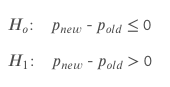With a confidence level of 95%, our alpha value (𝛼) is 0.05. That means we hope 95% different from the conversion of our old design. We can reject the null hypothesis if the p-value is less than the 0.05 alpha value.

## Analysis in PySpark

### Load the data into Spark

Let's load data and preprocess data.

``df = read("ab_test.ab_testing_data")``

### Clean up the data

We found that there are duplicate data.  We can eliminate the duplicates using PySpark.

``df_dedup = df.dropDuplicates(['user_id'])``

### Calculate the summary of controlled and treatment group

Since the data is available at a very detailed level, we will perform the summarization using PySpark.

``````df = spark.sql('''
select  count(case when `group` = 'control' then user_id else null end) as control_count,
count(case when `group` = 'treatment' then user_id else null end) as treatment_count,
sum(case when `group` = 'control' then converted else 0 end) as control_successes,
sum(case when `group` = 'treatment' then converted else 0 end) as treatment_successes,
count(user_id) as total_count
from    AB_TEST
''')``````

### ZTest PySpark UDF

The A/B Test for this use case is a proportion test and the standard way to calculate the proportion test is using z-test.

However, we did not find a PySpark library for calculating the z-test.

In this case, we create PySpark UDF that will call the Python library statsmodels.stats.proportion to calculate the metrics.

Create PySpark UDF has three steps:

1. Create a data frame: This is the data that will pass to the UDF as the input
2. Create a Python function: The logic will be implemented in Python that accepts that the data from each row
3. Convert a Python function to PySpark UDF: This step is to let Spark knows that the Python function is a UDF and thus will be passed to Spark cluster for execution.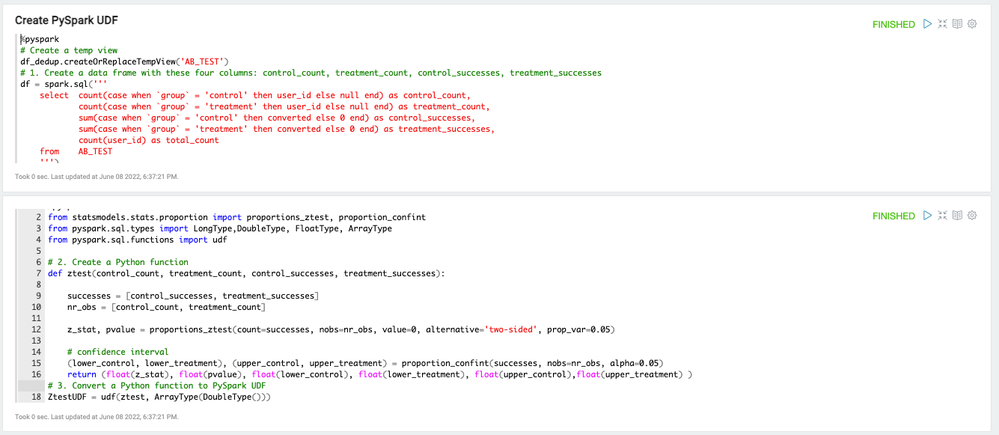### Call the PySpark UDF

After the UDF is created, we can use the UDF in a PySpark DataFrame using the withColumn clause and generate the result.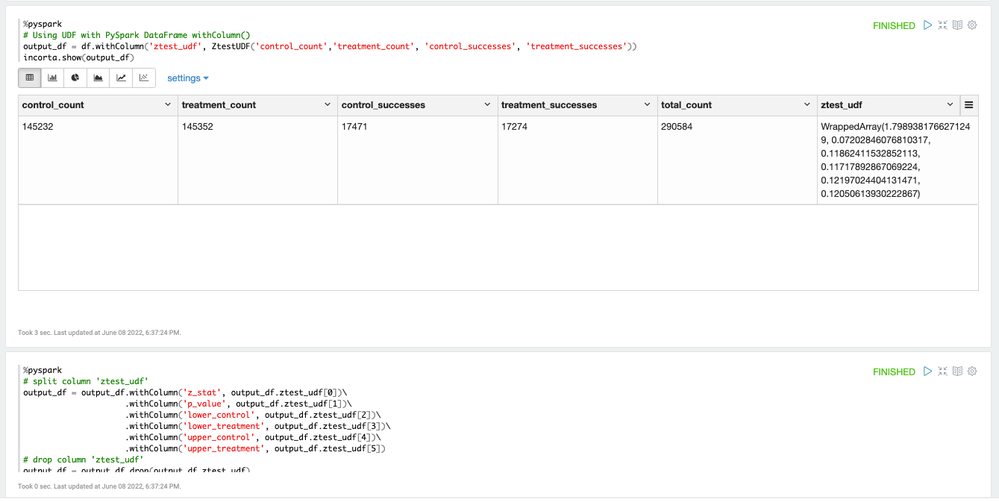### Convert the Resulted Array to DataFrame columns

The ZTest UDF returns the test result metrics in a Spark array.  In order to show and store the data with Incorta, we convert the data to individual columns.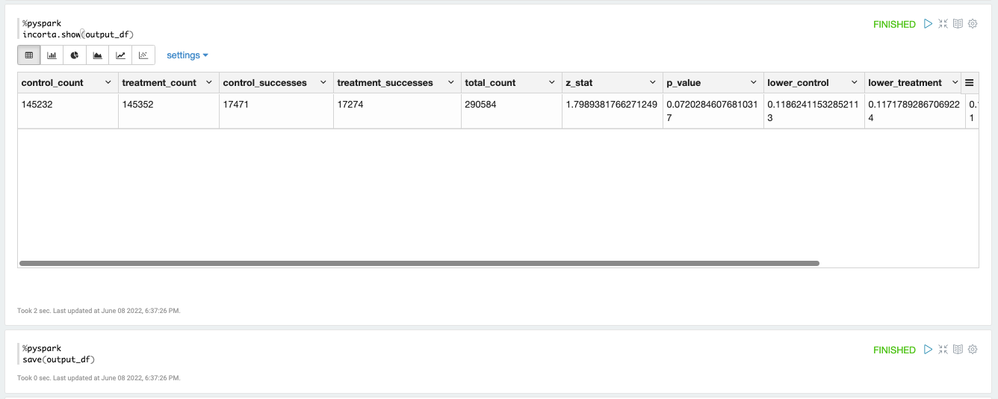### Conclusion

Based on the p-value of 0.072 which is much higher than our alfa(0.05), we cannot reject the null hypothesis. That means our new page design has the same similar effect as the old page.

## Sample Codes

``````# Load data
incorta.show(df)
# check user if sampled multiple times
df_dedup = df.dropDuplicates(['user_id'])
df.count(), df_dedup.count()
# Create a temp view
df_dedup.createOrReplaceTempView('AB_TEST')
# 1. Create a data frame with these four columns: control_count, treatment_count, control_successes, treatment_successes
df = spark.sql('''
select  count(case when `group` = 'control' then user_id else null end) as control_count,
count(case when `group` = 'treatment' then user_id else null end) as treatment_count,
sum(case when `group` = 'control' then converted else 0 end) as control_successes,
sum(case when `group` = 'treatment' then converted else 0 end) as treatment_successes,
count(user_id) as total_count
from    AB_TEST
''')
from statsmodels.stats.proportion import proportions_ztest, proportion_confint
from pyspark.sql.types import LongType,DoubleType, FloatType, ArrayType
from pyspark.sql.functions import udf

# 2. Create a Python function
def ztest(control_count, treatment_count, control_successes, treatment_successes):

successes = [control_successes, treatment_successes]
nr_obs = [control_count, treatment_count]

z_stat, pvalue = proportions_ztest(count=successes, nobs=nr_obs, value=0, alternative='two-sided', prop_var=0.05)

# confidence interval
(lower_control, lower_treatment), (upper_control, upper_treatment) = proportion_confint(successes, nobs=nr_obs, alpha=0.05)
return (float(z_stat), float(pvalue), float(lower_control), float(lower_treatment), float(upper_control),float(upper_treatment) )
# 3. Convert a Python function to PySpark UDF
ZtestUDF = udf(ztest, ArrayType(DoubleType()))
# Using UDF with PySpark DataFrame withColumn()
output_df = df.withColumn('ztest_udf', ZtestUDF('control_count','treatment_count', 'control_successes', 'treatment_successes'))
incorta.show(output_df)
# split column 'ztest_udf'
output_df = output_df.withColumn('z_stat', output_df.ztest_udf)\
.withColumn('p_value', output_df.ztest_udf)\
.withColumn('lower_control', output_df.ztest_udf)\
.withColumn('lower_treatment', output_df.ztest_udf)\
.withColumn('upper_control', output_df.ztest_udf)\
.withColumn('upper_treatment', output_df.ztest_udf)
# drop column 'ztest_udf'
output_df = output_df.drop(output_df.ztest_udf)
incorta.show(output_df)
save(output_df)``````

Best Practices IndexJust here to browse knowledge? This might help!

Contributors
Version history
Last update:
‎07-31-2022 05:17 PM
Updated by: